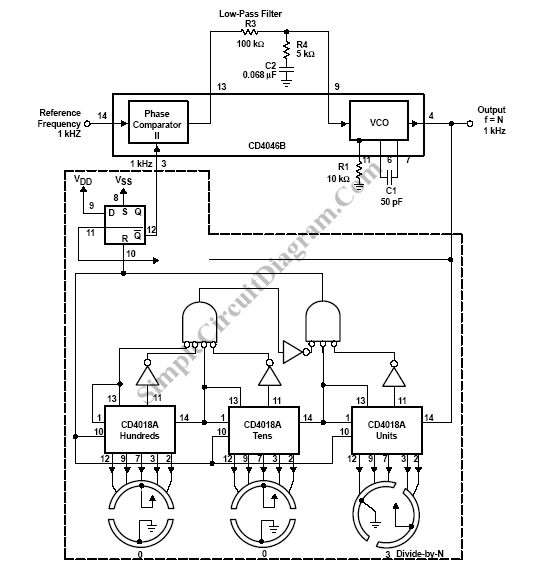# Frequency Synthesizer

A frequency-selective frequency multiplier can be construct with a PLL system by inserting frequency divider inside the feedback between the phase detector input and the VCO output. Figure below shows the schematic diagram of low-frequency synthesizer with a programmable three decades divider circuit.The frequency-divider modulus N have value between 3 to 999 with single steps increment. In locked condition, the comparator and signal are at same frequency that f=N*1kHZ.So we have a frequency synthesizer with 3KHZ to 999 KHZ range with 1-KHZ increment, which can be programed by the switch position of the divide-by-n counter.

This circuit uses phase comparator II because a frequency synthesizer shouldn’t lock on harmonics of the signal-input reference frequency. We can’t use phase comparator I because it does lock on harmonics. Phase comparator II correspond to this application because the active factor of the output of the divide-by-n frequency divider is not 50%. The VCO is set by Phase comparator II,to cover a range of 0 MHz to 1.1 MHz. This application have  two-pole of the LPF. To faster locking for step changes in frequency this application have tag-lead filter. [Schematic diagram source: Texas Instruments Application Report]